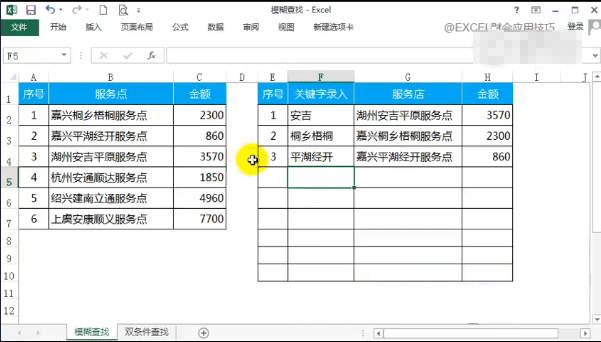Excel VLOOKUP+IF 模糊匹配的经典公式=IF(\$F4="","",VLOOKUP("*"&\$F4&"*",\$B:\$C,COLUMN(A1),0))

=IF(\$F4="","",LOOKUP(1,0/FIND(\$F4,\$B\$2:\$B\$7),B\$2:B\$7))